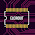# OCL Power Amplifier 50Watt

Power amplifier circuit with PCB and component list about 50W OCL Power Amplifier

Power Amplifier OCL 50 Watt is a power amplifier that is now very popular and widely used for audio in homes. Besides the price is relatively affordable, the sound quality was arguably tolerable for audio lovers. We will share a little about the circuit and component list that is in Power Power Amplifier OCL 50 Watt.

Circuit Diagram:
Components list:
Resistors
R1=30K
R2=2K2
R3=100R
R4=15K
R5=5K6
R6=100R
R7=560R
R8=30K
R9=30K
R10=2K2
R11=2K2
R12=560R
R13=560R

Capacitors
C1=100N
C2=220N
C3=100uF
C4=4n7
C5=100pF
C6=100u

Diodes
D1=9V Zener
D2=1N4007
D3=1N4007
D4=1N4007

Transistors
Q1=A1015
Q2=A1015
Q3=D438
Q4=TIP42
Q5=TIP41
Q6=TIP3055
Q7=TIP2955

Power Supply: 35V DC Symmetrical
Output Power: 50Watt RMS

PCB Layout Design

## 22 comments for "OCL Power Amplifier 50Watt"

1.All Resistors w????

1.u can use 1/4W or 1/2W sir

2.D438 matching Transistors

3.D438 matching Transistors

1.D400 or you can use BD139

4.All Resistors w????

1.1/4W or 1/2W

5.6.Sir help 5200 and 1943 using 300w amplifier BCP design send sir please

7.Sir help 5200 and 1943 using 300w amplifier BCP design send sir please

8.1.Okay gopal youre wellcome

9.It is very useful for me to have gathered some important information from these sources.

goldenslot

สูตรบาคาร่า

สมัครแทงบอล

10.hi what is the pcb dimension sir

11.what's the pcb dimension sir pls thanks

1.50mm x 60mm PCB sizer

2.12.Sir what is the size of PCB

13.what is the size of PCB

14.mau nanya kang wahyu untuk GND dari psu ke kit powernya kemana ya kang soalnya ga ada gnd nya

15.Why you design with bjt , not fets? Shouldnt they be more linear and efficiant?

16.BD488 IS PNP AND BD 139 IS NPN ????